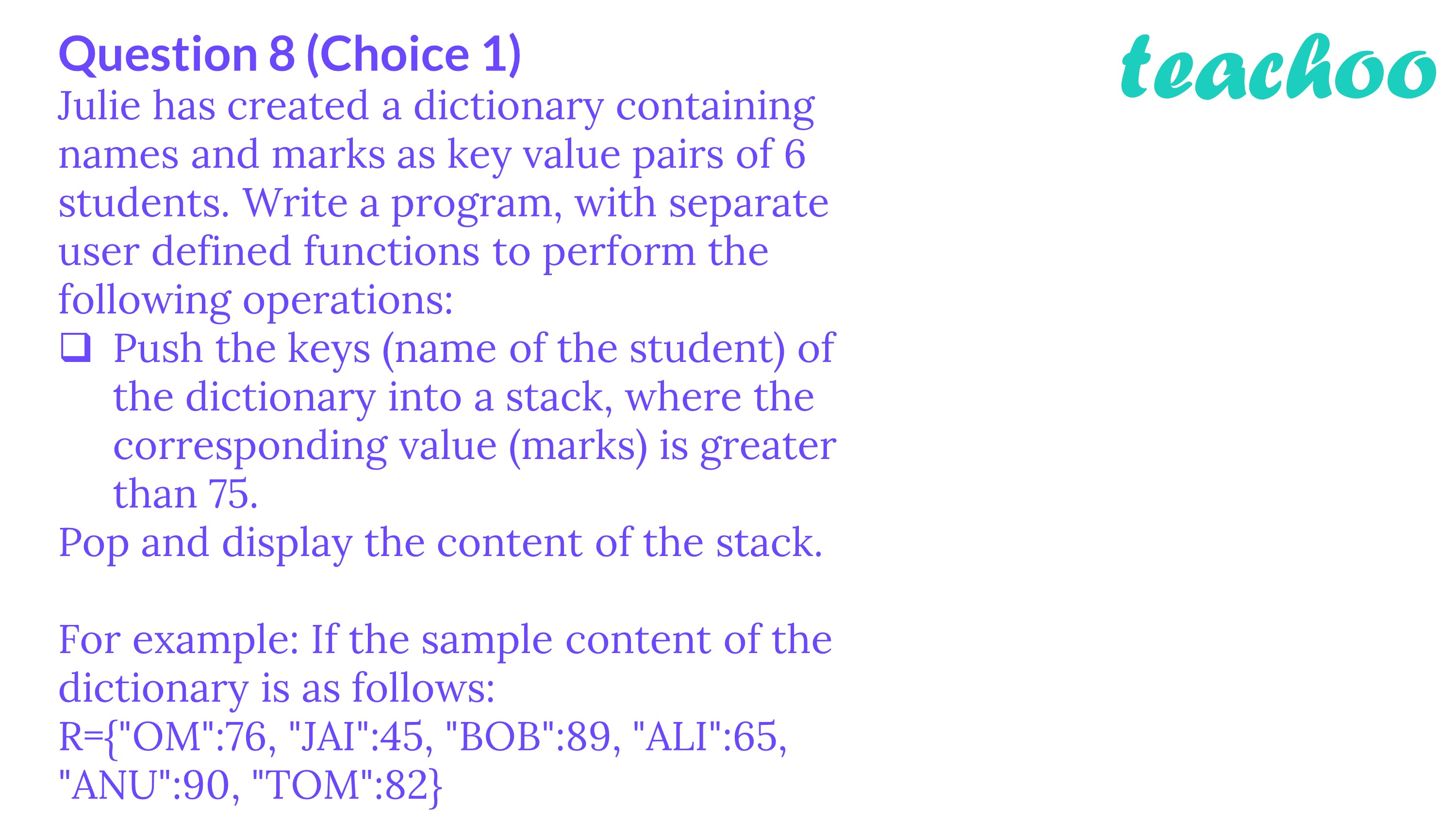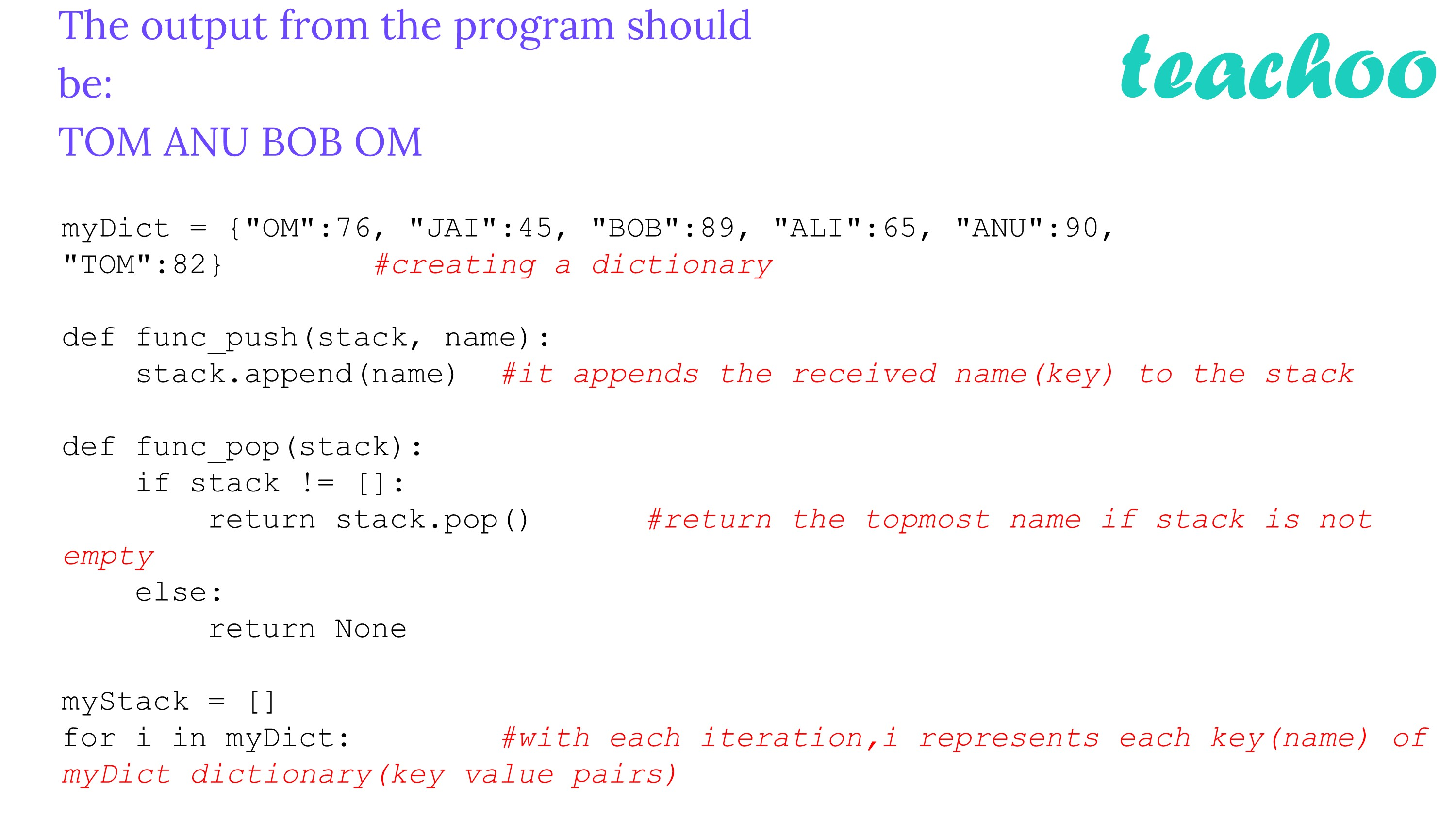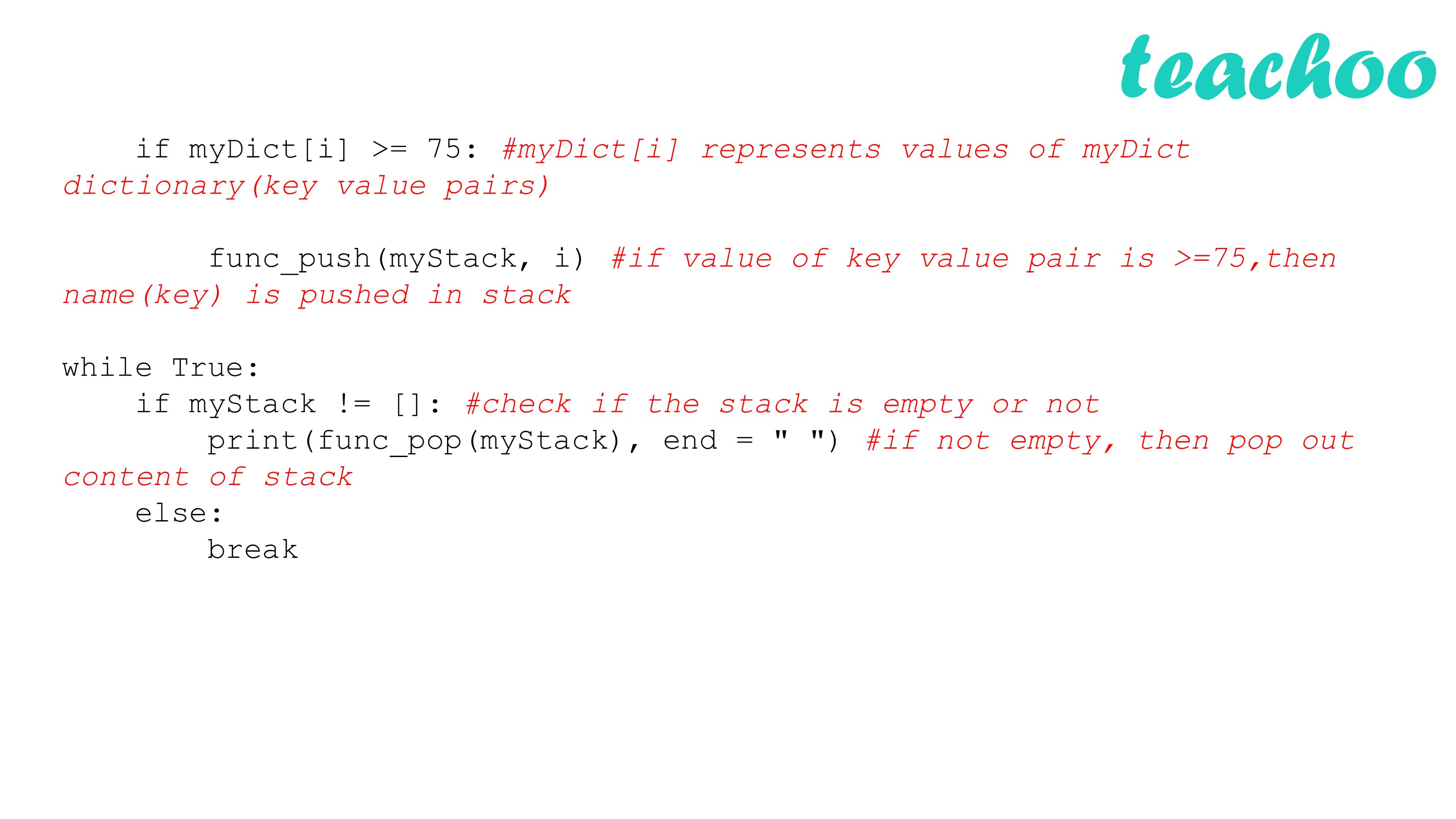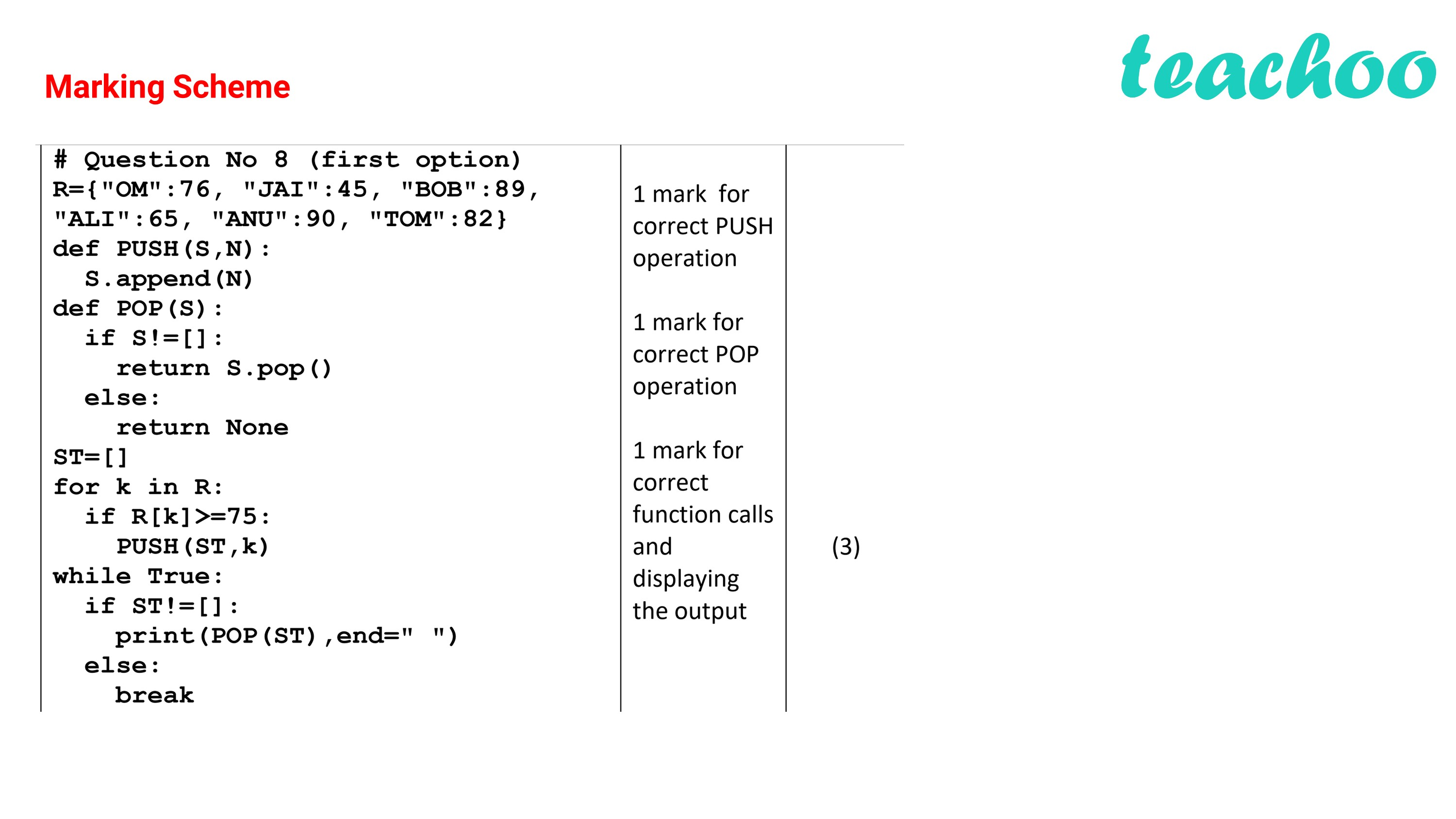CBSE Class 12 Sample Paper for 2022 Boards [Term 2] - Computer Science

Computer Science - Class 12
Solutions to CBSE Sample Paper - Computer Science Class 12

## The output from the program should be: TOM ANU BOB OMProgram:

``` myDict = {"OM":76, "JAI":45, "BOB":89, "ALI":65, "ANU":90, "TOM":82}        #creating a dictionary ```

``` def func_push(stack, name): ```

```     stack.append(name)      #it appends the received name(key) to the stack ```

``` def func_pop(stack): ```

```     if stack != []: ```

```         return stack.pop()      #return the topmost name if stack is not empty ```

```     else: ```

```         return None ```

```       ```

``` myStack = [] ```

``` for i in myDict:        #with each iteration,i represents each key(name) of myDict dictionary(key value pairs) ```

```     if myDict[i] >= 75:     #myDict[i] represents values of myDict dictionary(key value pairs) ```

```   ```

```         func_push(myStack, i)       #if value of key value pair is >=75,then name(key) is pushed in stack ```

```   ```

``` while True: ```

```     if myStack != []:       #check if the stack is empty or not ```

```         print(func_pop(myStack), end = " ")     #if not empty, then pop out content of stack ```

```     else: ```

```         break ```

Output:

``` TOM ANU BOB OM ```

``` ```

Learn in your speed, with individual attention - Teachoo Maths 1-on-1 Class